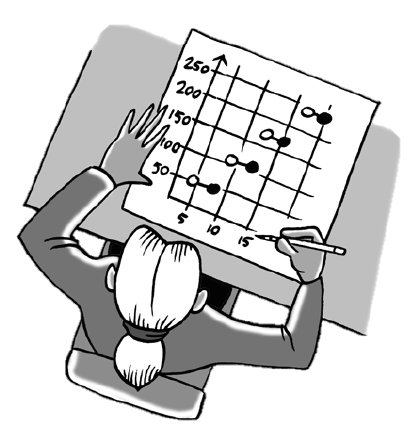### Home > CCA2 > Chapter 2 > Lesson 2.1.1 > Problem2-4

2-4.Jamilla was moving to a new city. She researched the rates charged by the local utility company for water. She found the listing of charges below. She expects that her family may use up to $1,000$ cubic feet of water each month.

• $\12.70$ monthly service fee

• First $300$ cubic feet of water used: $\3.90$ per $100$ cubic feet, or fraction thereof

• After the first $300$ cubic feet: $\5.20$ per $100$ cubic feet, or fraction thereof

1. Sketch a graph of the cost of Jamilla’s possible water usage in one month. Be sure to consider what the cost would be for partial units such as $220$ or $675$ cubic feet of water.

This will be a step graph. What is the cost if no water is used, but there is service?

When sketching this graph, note that the price jumps from $\3.90$ to $\5.20$ after $300$ cubic feet of water is used.

2. Is this graph a function? Why or why not?

For each $x$-value, is there exactly one $y$-value?

3. What are the domain and range of this graph?

Domain refers to the possible $x$-values, and range refers to the possible $y$-values.